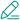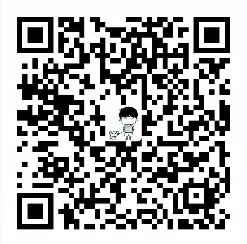#臭大佬

### 深入理解计算机系统之四 -- 指针

C语言里，变量存放在内存中，而内存其实就是一组有序字节组成的数组，每个字节有唯一的内存地址。指针是一种保存变量地址的变量。

### 指针声明

``````int  *ip
char **cpp
``````

### NULL指针

``````#include "stdio.h"

int main()
{
int *p = NULL;
printf("p的地址为%p\n", p);

return 0;
}

// p的地址为(nil)
``````

### 数组与指针

``````T A[N]
``````

``````#include "stdio.h"
int main()
{
// 声明一个int类型的数组，这个数组有10个元素
int a = {1, 2, 3, 4, 5, 6, 7, 8, 9, 0};
// 声明int类型的指针变量
int *p;
int *p1;
int *p2;
// 声明一个int类型的变量
int sub;
// 对指针进行初始化，p将指向数组 a 的第 1 个元素 a
p = &a;
// 数组类型的变量或表达式的值是该数组第 1 个元素的地址，且数组名所代表的的就是该数组第 1 个元素的地址，所以：p = a;
printf("a的地址为：%p\n", a);
printf("a的地址为：%p\n", &a);
printf("p的地址为：%p\n", &p);
// 打印指针 p 的地址，并不是指针所指向的地方的地址
p1 = &a;
p2 = &a;
sub = p2 - p1;
printf("sub=%d\n", sub);
// sub=6
p += 2;
printf("*(p+2)的值为：%d\n", *p);
// *(p+2)的值为：3
// 输出结果为 3，*(p+2)指向了 x
return 0;
}
``````

### 指针与函数

``````#include "stdio.h"
// 参数为普通的 int 变量
void swap1(int a, int b)
{
int temp;
temp = a;
a = b;
b = temp;
}
// 参数为指针，接受调用函数传递过来的变量地址作为参数，对所指地址处的内容进行操作
void swap2(int *a, int *b)
{
// 最终结果是，地址本身并没有改变，但是这一地址所对应的内存段中的内容发生了变化，即x,y的值发生了变化
int temp;
temp = *a;
*a = *b;
*b = temp;
}
int main()
{
int x = 1, y = 2;
swap1(x, y);              // 将 x,y 的值本身作为参数传递给了被调函数
printf("%d %5d\n", x, y); // 输出结果为：1     2
swap2(&x, &y);            // 将 x,y 的地址作为参数传递给了被调函数，传递过去的也是一个值，与传值调用不冲突
printf("%d %5d\n", x, y); // 输出结果为：2     1
return 0;
}
``````

{{ comment.content }}@ {{ child.answered_user_name }}:{{ child.content }}• 微信• 如果你觉得本站很棒，可以通过扫码支付打赏哦！

•微信收款码
•支付宝收款码